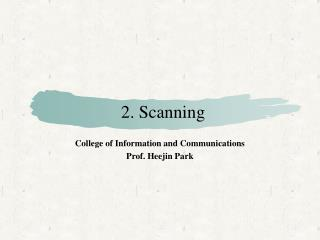DownloadDownload Presentation2. Scanning

# 2. Scanning

Download Presentation## 2. Scanning

- - - - - - - - - - - - - - - - - - - - - - - - - - - E N D - - - - - - - - - - - - - - - - - - - - - - - - - - -
##### Presentation Transcript

1. 2. Scanning College of Information and Communications Prof. Heejin Park

2. Overview • The scanning process • Regular expressions • Finite Automata • DFA • NFA

3. The scanning process • a[index] = 4 + 2 a /[ / index /] / = / 4 / + / 2

4. The scanning process • Tokens • Reserved words • IF, THEN, ELSE,… • if, then, else, … • Special symbols • PLUS, MINUS, … • +, - • tokens for multiple strings • NUM • 123, 456, …. • ID • a, index

5. The scanning process • Data structures for tokens typedef struct { TokenType tokenval; char * stringval; int numval; } TokenRecord; typedef struct { TokenType tokenval; union { char * stringval; int numval; } attribute; } TokenRecord;

6. The scanning process • Scanning and parsing are mixed together. • getToken(void); • This function returns the next token one by one.

7. The scanning process • Representing lexemes • enumeration? • {if, then, else, … +, -, …, 0, 1,2, … a, b, c, …} • It may be appropriate for reserved words and special symbols. • Not appropriate for numbersand identifiers. • Inefficient • Representing using regular expression

8. Regular expressions • Definitions • symbols: characters • a, b, c, +, -, ... • alphabet (Σ): set of legal symbols • {A,B, C, …, Z, a, b, c, …, z} • strings: concatenation of symbols • I am a boy • A regular expressionr represents • a set of strings that is called the language generatedbyr, i.e., L(r).

9. Regular expressions • Regular expressions • A symbol can be a regular exp. • a: L(a) = {a}, b: L(b) = {b}, … • ε: L(ε) = {ε}, Φ: L(Φ) = {} • Choice among regular exps is a regular exp. • r|s: L(r|s) = L(r) U L(s) • example) • L(a|b) = {a} U {b} = {a,b} • L(a|b|c|d) = {a,b,c,d}

10. Regular expressions • Regular expressions • Concatenation of regular exps is a regular exp. • rs: L(rs) = L(r)L(s) • example) L(ab) = {ab} • Repetition of a regular exp is a regular exp. • r*: L(r*) = {ε} UL(r ) UL(rr ) UL(rrr ) … • example) L(a*) = {ε, a, aa, aaa, …}

11. Regular expressions • Further examples • (a|b)c • L((a|b)c) = L(a|b)L(c) = {a,b}{c} = {ac, bc} • (a|bb)* • L((a|bb)*) = {ε, a, bb, aa, abb, bba, bbbb, ...} • Precedence of operations • * > · > | • a|bc*: L(a|bc*) = L(a)U L(b)L(c)* • Names • (0|1|2|…|9)(0|1|2|…|9)* • It can be rewritten as digit digit* where digit = 0|1|2|…|9.

12. Regular expressions • Examples • The set of all strings over {a,b,c} containing exactly one b. • (a|c)*b(a|c)* • The set of all strings over {a,b,c} containing at most one b. • (a|c)* | (a|c)*b(a|c)* • (a|c)*(b|ε)(a|c)* • The set of all strings over {a,b}consisting of a single b surrounded by the same number of a’s. • {b, aba, aabaa, …} • impossible

13. Regular expressions • Extensions to regular expressions • + : one or more repetitions • r+ = rr* • (0|1|2|…|9)(0|1|2|…|9)*  (0|1|2|…|9)+ • . : any symbol in the alphabet • .*b.* • - : a range of symbols • a|b|c [abc] • a|b|…|z [a-z] • [a-zA-Z]

14. Regular expressions • Extensions to regular expressions • ~, ^: any symbol not in a given set • ~(a|b|c) or [^abc]: a character that is not either a or b or c • ?: optional subexpressions • natural = [0-9]+ • signedNatural = natural | +natural | - natural  signedNatural = (+|-)?natural

15. Regular expressions for PL tokens • Reserved words • reserved = if | while | do | … • Special symbols • Identifiers • letter = [a-zA-Z] • digit = [0-9] • identifier = letter(letter|digit)*

16. Regular expressions for PL tokens • Numbers • nat = [0-9]+ • signedNat = (+|-)? nat • number = signedNat(“.” nat)? (E signedNat)? • Comments • {this is a Pascal comment} • -- this is an Ada comment • /* this is a C comment */

17. Regular expressions for PL tokens • Comments • {this is a Pascal comment} • {(~})*} • -- this is an Ada comment • --(~newline)* • /* this is a C comment */ • ba …. ab where b = / and a = *. • ba (b*(a*~(a|b)b*)*a*) ab

18. Regular expressions for PL tokens • Ambiguity • Isif a keyword or an identifier? • Is temp an identifier temp or identifiers te and mp? • Disambiguating rules • Keyword is preferred to identifiers. • if is a keyword. • principle of longest substring • temp is an identifier temp.

19. Regular expressions for PL tokens • Token delimiters • White space • whitespace = (blank | tab | newline | comment)+ • do if, do/**/if • Characters that are unambiguously part of other tokens. • xtemp=ytemp

20. Regular expressions for PL tokens • lookahead and backtrack • single-character lookahead • xtemp=ytemp • backtrack (more than single-character lookahead) • FORTRAN • DO99I=1,10 (loop) • DO99I=1.10 (assignment)